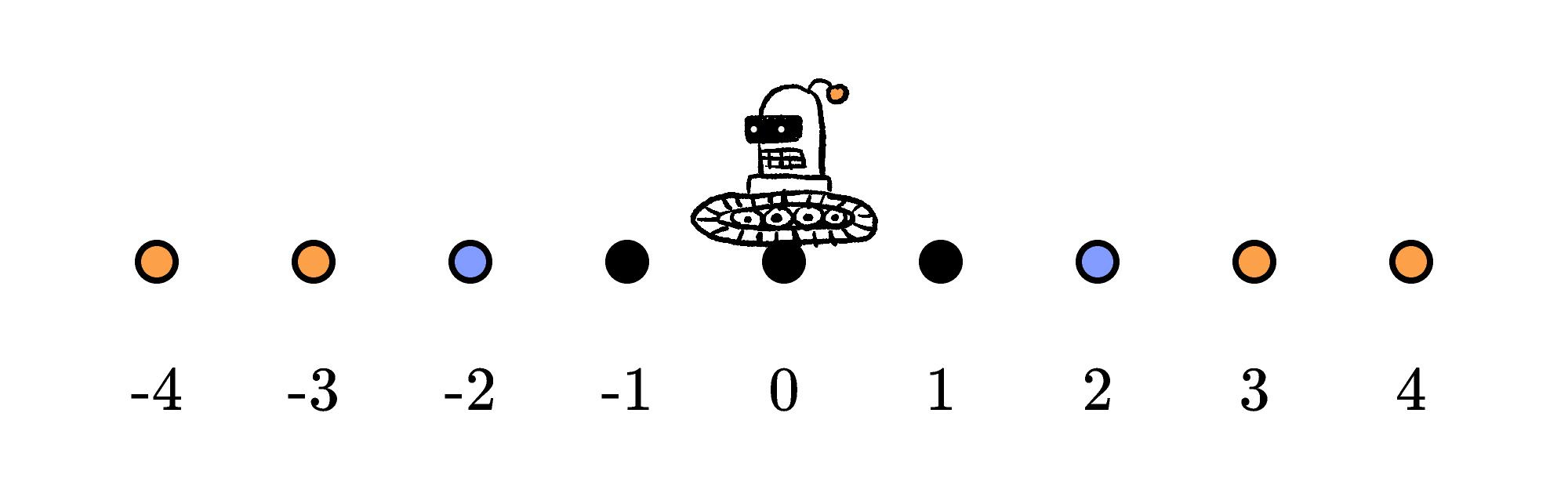Back

## Wandering Robots

Today we'll try to unpack a general strategy for working on probability problems, specifically ones where many events happen in sequence. Or you can decide to go straight to the challenge.

Here's the strategy:

Instead of thinking about a series of probabilistic events happening to one agent or object, imagine dozens or even thousands of agents and apply the probabilities of different events as a ratio to figure out how many agents you can expect to have each possible sequence of experiences.

The number of agents that have some specific experience, divided by the total number of agents, is the probability that a single agent will have that experience.

This problem-solving strategy doesn't eliminate the mathematical steps of solving probability problems, but it will let you work with whole numbers instead of fractions. Just be sure to pick a large enough number of agents that you don't have to deal with one-third of one agent having one experience while the other two-thirds of the agent has another. In this problem, using an initial number of agents that's a large power of two is particularly effective, since we repeatedly split this group in half.

In today's challenge, a hopping robot moves between several dots that are colored, not numbered. But let's assign each dot a number to make it easier to talk about the robot's motion.Also, while there are only nine dots in this image, you can imagine that the row goes out forever in both directions and that the numbering keeps up accordingly. Now let's look for some patterns in how the robot moves.

We can think of hopping one dot to the right as adding $1$ to the robot's position, while a move to the left is subtracting $1.$ For example, the sequence left, right, left, left would put the robot at dot $0 - 1 + 1 - 1 - 1 = -2.$

One important observation that we can support with this arithmetic perspective is that the number of hops left vs. the number of hops right is all that you need to know to determine the robot's final position. The order in which the robot makes those hops doesn't matter at all in determining the robot's final destination. For example, the sequence left, left, left, right would also put the robot at dot $0 - 1 - 1 - 1 + 1 = -2.$ This observation can definitely help you solve today's challenge efficiently.

However, what if we don't just care about the robot's destination, what if we care about what dots it visits en-route? One interesting and challenging behavior that we can study is the chance a randomly hopping robot has of returning to dot $0$ at least once in the middle of its hopping sequence. As a warm-up to today's challenge, let's look at a robot that makes a six-hop journey.

So that we don't have to talk about everything in terms of fractions, let's think about starting with $2^6 = 64$ robots at dot $0,$ and study what happens if, at each chance they are given to hop, half of the robots on each dot hop one dot left and the other half hop one dot right. Additionally, if a robot ever returns to dot $0,$ we'll "recapture" it, stop tracking its movement, and add it to the tally in the second-to-lowest row of the chart below. This will ensure that we don't count one robot that returns to $0$ twice as if it was two robots, each returning to $0$ once.

$($Although... If we wanted to start a "tag and release" program, we could use a three-dimensional chart to track robots that are captured at $0$ multiple times.$)$

Here's the distribution of robots on each dot if you track each of $64$ robots for either $6$ hops or until it's recaptured at dot $0:$

 Position After $N$ Hops: $0$ $1$ $2$ $3$ $4$ $5$ $6$ $-6$ $1$ $-5$ $2$ $\color{#EC7300}-4$ $4$ $4$ $\color{#EC7300}-3$ $8$ $6$ $\color{#3D99F6}-2$ $16$ $8$ $5$ $-1$ $32$ $8$ $4$ $\enspace 0$ $64$ $32$ $8$ $4$ $\enspace 1$ $32$ $8$ $4$ $\enspace \color{#3D99F6}2$ $16$ $8$ $5$ $\enspace \color{#EC7300}3$ $8$ $6$ $\enspace \color{#EC7300}4$ $4$ $4$ $\enspace 5$ $2$ $\enspace 6$ $1$ Total count recaptured at $0:$ $0$ $0$ $32$ $32$ $40$ $40$ $44$ Total count still wandering: $64$ $64$ $32$ $32$ $24$ $24$ $20$

So, after up to $6$ hops, $44$ out of $64$ robots will have been recaptured at $0$ and only $20$ of the robots will remain wandering.

Correspondingly, that means the chance of any individual robot returning at least once to $0$ over the course of a $6$-hop journey is $\frac{44}{64} = \frac{11}{16} \approx 69\%.$ If you want to explore this more, try using a spreadsheet or write a program that shows what happens when you let loose thousands or tens of thousands of robots and let them wander for dozens or hundreds of hops.

In today's challenge, the robot doesn't get captured on any dot, but you can use a similar chart to figure out the chance of a robot ending a four-hop journey on either $-2$ or $2.$

# Today's Challenge

Every time it moves, this robot either hops exactly one dot to the left or one dot to the right.If the robot starts in the center of this pattern, what is the probability that, after four hops, it's positioned on top of a blue dot?×